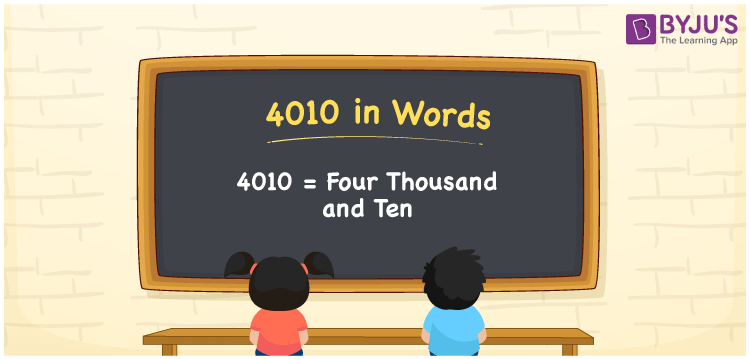# 4010 in Words

4010 in words is Four Thousand and Ten. In Mathematics, the number 4010 is a natural number and it has four digits. For example, the cost of 10 books is Rs. 4010. Generally, the numbers are converted into words using the place value system. In this article, we have discussed the spelling of 4010 in English words and its procedure in detail.

 4010 in Words: Four Thousand and Ten. Four Thousand and Ten in Numerical Form: 4010.

## 4010 in English Words## How to Write 4010 in Words?

Go through the below table which represents the place values of the number 4010.

 Thousands Hundreds Tens Ones 4 0 1 0

The expanded form of 4010 is as follows:

= 4 × Thousand + 0 × Hundred + 1 × Ten + 0 × One

= 4 × 1000 + 0 × 100 + 1 × 10 + 0 × 1

= 4000 + 10

= 4010

= Four thousand and ten

Hence, 4010 in words is four thousand and ten.

4010 in words – Four thousand and ten

Is 4010 an odd number? – No

Is 4010 an even number? – Yes

Is 4010 a perfect square number? – No

Is 4010 a perfect cube number? – No

Is 4010 a prime number? – No

Is 4010 a composite number? – Yes

## Frequently Asked Questions on 4010 in Words

Q1

### How to spell 4010 in words?

4010 in words is four thousand and ten.

Q2

### Simplify 4000 + 10, and express it in words.

Simplifying 4000 + 10, we get 4010. Hence, 4010 in words is four thousand and ten.

Q3

### Is 4010 an even number?

Yes, 4010 is an even number.